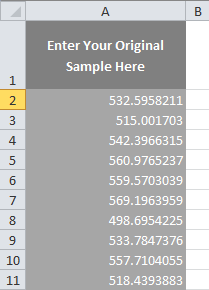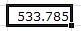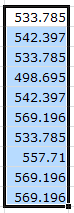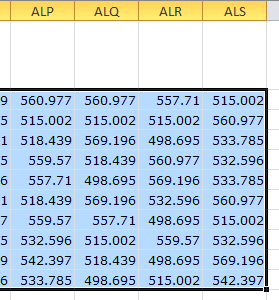# Bootstrapping Statistics & Confidence Intervals, Tutorial

If you use applied statistics in your career, odds are you’ve used the Great Assumption Of Our Era, the assumption of the Normal distribution.

There are some good reasons for this. The Central Limit Theorem is usually thrown in there as a justification, and it works reasonably well for practical applications. But the Central Limit Theorem really counts on that convergence as n goes to infinity.

And while “infinity” might be closer to 40 in most practical applications, in the real world even a sample size of 40 often costs too much for real world budgets.

If you’re assuming your samples are coming from the Normal distribution, you might be making a mistake. This is especially likely if you’re trying to estimate less obvious statistics like medians, odds ratios, and so on.

(You can use this spreadsheet to test whether your data comes from a Normal distribution, but remember, if your sample isn’t large to begin with, a null result is meaningless.)

Here’s the truth. The only thing you really know about the population you’re trying to estimate is the sample itself. And it turns out that we can actually use this to our advantage using a method called the Bootstrap.

In fact, the Bootstrap method has been shown to be more accurate than traditional methods in many circumstances. It works when samples aren’t “well behaved” or when sample sizes are small (even as small as 8), and it’s much easier to use if the statistic you’re trying to calculate is…complicated.

Oh, and error propagation is built in too.

(Alright, before you get too excited, as with anything else, there are some circumstances where Bootstrapped statistics aren’t acceptable. I’ll let you know about them in this blog post.)

As usual, if you don’t want to go through the trouble of setting this up yourself, I’ve also uploaded a spreadsheet you can use to skip all the busy work. This spreadsheet calculates a Bootstrapped confidence interval for your mean, standard deviation, median, Q1, and Q3 based on 1000 resamples. You’ll need Excel 2007 or higher. (Feel free to edit the spreadsheet to do more resamples, I just don’t want to be the one who crashes your computer…and I might anyway, so manual calculation mode is recommended.)

## What is the Bootstrap Method?

The Bootstrap method for finding a statistic is actually intuitively simple, much simpler than more “traditional” statistics based on the Normal distribution. The only reason it didn’t get used first is because it requires a lot of computation.

Here’s how it works.

You start by taking a real sample like, say, 10 items off of a production line.

The next thing you do, is you take random samples, with replacement, of that original sample. (Meaning you are free to draw the same result more than once.) These new, simulated samples should be the same size as the original sample.

Then you want to take “a lot” (as in, however many it takes to converge) of these simulated samples.

For each of these simulated samples, you calculate the statistic you care about, like your average or your standard deviation.

Now what you have is a representative sample of your statistic.

For example, want to build a 95% confidence interval for your population average? Just take 1,000 random samples from your original sample, and find the average for each of those simulated samples.

Then just put your resampled averages in order, pick the 25th result for your lower bound, and pick the 975th result for your upper bound.

## Is There Ever a Case Where it’s Not Okay to Bootstrap?

Yes.

While bootstrapping relies on fewer assumptions than more traditional statistics, is more accurate in many circumstances, requires smaller sample sizes, is easier to implement for complicated statistics, and is safer to use, you can’t use it in every situation. Here are a few examples, as mentioned in this Rutgers University paper:

• Bootstrapping maximums or minimums of a population isn’t possible (though, for comparison, the normal distribution assumes there is no such thing anyway, while the Bootstrap method does not.)
• You can’t use it to estimate a population mean or standard deviation if the population in question has infinite variance. (Yes, there are weird distributions like this. Again, the assumption of normality has the same problem.)
• You can’t bootstrap eigenvalues if those eigenvalues have multiplicity.
• You can’t estimate a population median if the population just happens to be discontinuous at the median.

It’s also important to understand that the bootstrap method still assumes that each sample is identically and independently distributed. This mathematical abstraction is customary in statistics, when the sad reality is that real distributions often do change over time, and taking a sample sometimes does influence the distribution.

Sorry, you’ll just have to hope that the influence of these factors is small enough not to matter. That’s how it goes with applied statistics.

## How to Set Up a Bootstrap in Excel (AKA, Enough Rambling)

Alright, here goes.I start by pasting the data in a column, starting in cell A2.

Next, I start taking resamples from this initial sample. I start off doing this by heading over to cell H2 and entering the formula:

=IF(ISBLANK(\$A2),””,INDEX(\$A:\$A,RANDBETWEEN(2,COUNTA(\$A:\$A)),1))

Now, this formula’s a bit more complicated than what you would necessarily need if you were setting this up yourself, and here’s why. The purpose of the IF statement is to only take a resample point from column A if the cell actually contains a number. If it’s blank, I don’t want it to take a sample. This is so you don’t have to resize the spreadsheet to fit your sample.

Anyway, the action is happening here:

INDEX(\$A:\$A,RANDBETWEEN(2,COUNTA(\$A:\$A)),1)

INDEX() returns a number from a specific row and column, which we can specify using formulas.

The first argument in the INDEX() function is for the reference, which should be your original sample. In this case, I’m using the entire column A.

The second argument in INDEX() is for the row. We need to choose a random row in order to take a random resample point from our original sample.

To do this, we use the RANDBETWEEN() function, which returns a random number between two values (including the first and last numbers that you specify). The first argument in RANDBETWEEN() is the smallest number you would ever want the function to return. I entered 2 here because the data starts on row 2.

The second argument in RANDBETWEEN() is the biggest number you would ever want the function to return. Here I use the COUNTA function to count the number of cells in column A that are not empty. (If you use this spreadsheet, you’ll need to make sure you don’t have any blank cells in your initial sample.) This returns the final row in our data column.

If you’re setting this up yourself, you could just specify the final row in your data column, rather than using the COUNTA function.

The final argument in the INDEX() function is the column number in your reference. Since all of the data is in one column, I just enter 1 here.

I get a result like this:Next, I grab the black box at the bottom right of this cell, and drag it down to cover all of the sample data. (Remember, our resamples need to be the same size as our original sample.) In my case, I drag it down to row 1001, because I want to be able to accommodate an initial sample size as high as 1,000. The IF() argument I used earlier prevents any cells from populating below the final row of the initial sample.

So, now I have one initial resample:Of course, the key to Bootstrapping is taking many resamples. So the next thing I do is grab the black box at the bottom right of the bottom cell, and drag it over 1000 columns. In my case, this is from column H to column ALS. (You can briefly switch over to R1C1 style in order to view your column headings as numbers instead of letters.)Now I have 1,000 random resamples of the initial sample.

The next step is to find an estimator of our statistic for each one of these samples. For example, to find the sample mean, I simply use the formula:

=AVERAGE(H2:H1001)

…in column H. (In my case it goes to H1001 because, again, I want to be able to accommodate an initial sample size as high as 1000. The point is to simply take the average from your first resample.)

Then I simply drag this formula all the way over to column ALS to get 1,000 resample averages. Put another way, I generate 1,000 estimates of the population average.

### Finding Confidence Intervals Using the Percentile Bootstrap Method

So, the method I’m about to share for finding your confidence intervals, called the percentile method, is the most intuitive and widely used, but I should stress that it’s not necessarily the best.

While this method still tends to outperform the assumption of normality or using a t-distribution, even with small sample sizes, confidence intervals can still end up too narrow if the distribution in question has strong skewness or if sample sizes are very small.

An improvement on the percentile bootstrap method is called the Bias-Corrected and Accelerated Bootstrap (BCa), which is close to the state of the art (along with double, triple, etc. bootstraps), but setting it up in Excel wouldn’t be particularly practical.

With that disclaimer out of the way, finding a confidence interval using the percentile bootstrap method is very easy once you have a large number of resampled statistics to work with.

For example, to find the lower bound of the confidence interval for the mean on my spreadsheet, I used this formula:

=PERCENTILE(1002:1002,(1-\$C\$2)/2)

The first argument in the PERCENTILE function contains the data that you want to pull your percentile from. Here I used the 1002nd row, because that is where the resampled means are located.

The second argument contains the percentile you want to pull from the distribution. For example, if you wanted to find a 95% confidence interval, you would want to use 0.025 here. For the upper bound of the confidence interval, you would use 0.975.

In my case, cell C2 contains a confidence level that you choose. This is then used to find alpha, which is divided by 2 in order to find the percentile on this end of the tail that we want to chop off.

You can do the same thing with most statistics, with a few exceptions, as mentioned above.

## How Can I Do Bootstrapping in R?

This is a good place to start. As far as confidence intervals go, with the boot.ci() function, I would recommend paying most attention to type=”stud” (for Studentized) and type=”bca” (for Bias-Corrected and Accelerated) results, since these have been shown to be the most accurate in an academic comparison. The type=”perc” option is also worth paying attention to since it is the most widely-known bootstrap method, the one discussed in the tutorial above, and it is much faster to calculate for large sample sizes.

## Bootstrapping in SPSS

This PDF is an in-depth guide about bootstrapping and bootstrapped confidence intervals in SPSS. As mentioned above, the BCa option is the most accurate, but it can take a while longer to run if your samples are large, while the Percentile option is the most widely known option.

## SAS Code For Bootstrapping

There’s actually an SAS macro specifically for Bootstrapping that should make things simpler, but there’s also a tutorial here.

## How Does Bootstrapping Compare to the Jackknife Method?

The Bootstrapping method typically outperforms the Jackknife method, which is quite a bit older, but closely related. See this academic discussion:

It performs less well than the bootstrap in Tables 1 and 2, and in most cases investigated by the author (See Efron 1982), but requires less computation.

The Jackknife method is similar, but is based on producing resamples that leave out a single sample point. This means that the number of resamples should be equivalent to the number of sample points in your initial, real sample.

These days, there are very few cases where the Jackknife method is better than the Bootstrap method, which is why I’m not spending much time on this.

## Is it Valid to Use Bootstrapping On Binary Data?

Yes, and this is a great example of what makes the Bootstrap method so powerful. You can use it on odds ratios, variance in binary data, proportions, and pretty much anything else you might encounter in the real world.

## Is it Possible to Use the Bootstrap on Summary Data, Rather than Raw Data?

As you can probably guess, you need the raw sample data in order to perform a Bootstrap, since this is the only way you can have access to the empirical distribution and resample from it.

## Can You Use Bootstrapping in Regression and Multivariate Statistics?

Absolutely.

Essentially, all you need to do is construct a sample regression formula for reach resample. Then you can use this to construct confidence intervals for your regression coefficients. There are two approaches to accomplishing this, called the random-x resampling method and the fixed-x resampling method.

You can find an in-depth discussion here.

And yes, this works for logistic regression and other advanced regression techniques.

The same goes for multivariate statistics.

## Why Does the Bootstrap Method Work?

Oh boy, isn’t that a fun question?

Well, I’m not going to provide an advanced mathematical proof, but here’s my “good enough” (hopefully) explanation.

Here’s what I think trips people up. They find it strange that a small sample (say 10) can be used to generate a larger sample (say 1000 resamples of size 10), and that this larger sample is somehow going to provide more information about the population than the initial sample.

But actually, that’s not what’s happening at all.

We’re not using the resamples to learn something more about the population. We’re using resamples to understand more about the distribution of the sample statistic, and that information is contained within the original sample.

While a sample of size 10 can only tell us about 10 points from the original population, it can theoretically tell us where up to 92,378 of the sample statistics lie. (Don’t believe me? See for yourself. n=10, r=10, order doesn’t matter, and repetition is allowed.)

Since the initial samples were taken at random, these 92,378 possible sample statistics should also be taken randomly (ahem…”more or less”) from the initial distribution of the statistic, even though we don’t know what that distribution is.

So, while this distribution of resampled statistics isn’t identical to the original distribution of the statistic, it is (typically) representative and it is also very large. So, under most circumstances, unless what you’re trying to estimate is at (or very close to) the extremes of the distribution, you should be fine in treating it as though it were the distribution of the statistic.

In fact, another method of doing the bootstrap is to painstakingly generate every possible resampled statistic, and use that as our distribution. It’s just that we typically don’t do this because random resamples are “good enough,” as long as we take enough of them.